Importance: Medium ✭✭
 Author(s): Deza, Antoine Terlaky, Tamas Zinchenko, Yuriy
 Subject: Geometry » Polytopes
 Keywords: curvature polytope
 Posted by: deza on: September 30th, 2007
Conjecture   The order of the largest total curvature of the primal central path over all polytopes defined byinequalities in dimensionis.

Letdenote the total curvature of the central path corresponding to the linear optimization problem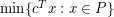. The quantitycan be regarded as the continuous analogue of the edge-length of the shortest path between a pair of vertices. Considering the largestover all possiblewe obtain the quantity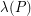, referred to as the curvature of a polytope. Following the analogy with the diameter, let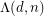be the largest total curvatureof the primal central path over all polytopesdefined byinequalities in dimension.

Holt and Klee~[HK] showed that, for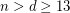, the conjecture of Hirsch is tight. We have the following continuous analogue of the result of Holt and Klee:

[DTZa]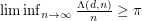, that is,is bounded below by a constant times.

The special case ofof the conjecture of Hirsch is known as the-step conjecture, and it has been shown by Klee and Walkup~[KW] that the-step conjecture is equivalent to the Hirsch conjecture. We have the following continuous analogue of the result of Klee and Walkup:

[DTZb] If the order of the curvature is less than the dimensionfor all polytope defined byinequalities and for all, then the order of the curvature is less that the number of inequalities for all polytopes; that is, iffor all, then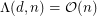.

## Bibliography

[DMS] J.-P. Dedieu, G. Malajovich and M. Shub: On the curvature of the central path of linear programming

*[DTZa] A. Deza, T. Terlaky and Y. Zinchenko: Polytopes and arrangements : diameter and curvature. Operations Research Letters (to appear).

[DTZb] A. Deza, T. Terlaky and Y. Zinchenko: The continuous d-step conjecture for polytopes. AdvOL-Report 2007/16, McMaster University (2007).

[HK] F. Holt and V. Klee: Many polytopes meeting the conjectured Hirsch bound. Discrete and Computational Geometry 20 (1998) 1--17.

[KW] V. Klee and D. Walkup: The-step conjecture for polyhedra of dimension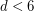. Acta Mathematica 133 (1967) 53--78.

* indicates original appearance(s) of problem.